OUTPUT Statement

OUTPUT <OUT= SAS-data-set> options ;

The OUTPUT statement creates a SAS data set that contains statistics computed by PROC FREQ. You specify which statistics to store in the output data set with the OUTPUT statement options. The output data set contains one observation for each two-way table or stratum, and one observation for summary statistics across all strata. For more information about the contents of the output data set, see the section Contents of the OUTPUT Statement Output Data Set.

Only one OUTPUT statement is allowed for each execution of PROC FREQ. You must specify a TABLES statement with the OUTPUT statement. If you use multiple TABLES statements, the contents of the OUTPUT data set correspond to the last TABLES statement. If you use multiple table requests in a TABLES statement, the contents of the OUTPUT data set correspond to the last table request.

Note that you can use the Output Delivery System (ODS) to create a SAS data set from any piece of PROC FREQ output. For more information, see the section ODS Table Names.

Also note that the output data set created by the OUTPUT statement is not the same as the output data set created by the OUT= option in the TABLES statement. The OUTPUT statement creates a data set that contains statistics (such as the Pearson chi-square and its p-value), and the OUT= option in the TABLES statement creates a data set that contains frequency table counts and percentages. See the section Output Data Sets for more information.

You can specify the following options in the OUTPUT statement:

OUT=SAS-data-set

names the output data set. If you omit the OUT= option, the data set is named DATAn, where n is the smallest integer that makes the name unique.

options

specify the statistics you want in the output data set. Table 36.7 lists the available options, together with the TABLES statement options needed to produce the statistics. You can output groups of statistics by using group options identical to those available in the TABLES statement, which include the AGREE, ALL, CHISQ, CMH, and MEASURES options. Or you can request statistics individually.

When you specify an option in the OUTPUT statement, the output data set contains all statistics from that analysis—the estimate or test statistic plus any associated standard error, confidence limits, p-values, and degrees of freedom. See the section Contents of the OUTPUT Statement Output Data Set for details.

If you want to store a statistic in the output data set, you must also request computation of that statistic with the appropriate TABLES or EXACT statement option. For example, you cannot specify the option PCHI (Pearson chi-square) in the OUTPUT statement without also specifying a TABLES or EXACT statement option to compute the Pearson chi-square test. The TABLES statement option ALL or CHISQ requests the Pearson chi-square test. If you have only one TABLES statement, the EXACT statement option CHISQ or PCHI also requests the Pearson chi-square test. Table 36.7 lists the TABLES statement options required to produce the OUTPUT data set statistics. Note that the ALL option in the TABLES statement invokes the CHISQ, MEASURES, and CMH options.

Table 36.7 OUTPUT Statement Options

Option

Output Data Set Statistics

Required TABLES Statement Option

AGREE

McNemar’s test, Bowker’s test, simple and

weighted kappas; for multiple strata, overall

simple and weighted kappas, tests for equal

kappas, and Cochran’s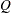AJCHI

Continuity-adjusted chi-square (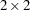tables)

ALL

CHISQ, MEASURES, and CMH statistics; N

BDCHI

Breslow-Day test (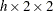tables)

CMH, CMH1, or CMH2

BINOMIAL

Binomial statistics for one-way tables

CHISQ

For one-way tables, goodness-of-fit test;

for two-way tables, Pearson, likelihood-ratio,

chi-squares, Fisher’s exact test (tables),

phi and contingency coefficients, Cramer’s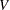CMH

Cochran-Mantel-Haenszel (CMH) correlation,

row mean scores (ANOVA), and general

association statistics; fortables, logit and

relative risks, Breslow-Day test

CMH1

CMH output, except row mean scores (ANOVA)

CMH or CMH1

and general association statistics

CMH2

CMH output, except general association statistic

CMH or CMH2

CMHCOR

CMH correlation statistic

CMH, CMH1, or CMH2

CMHGA

CMH general association statistic

CMHRMS

CMH row mean scores (ANOVA) statistic

CMH or CMH2

COCHQ

Cochran’s(tables)

CONTGY

Contingency coefficient

CRAMV

Cramer’sEQKAP

Test for equal simple kappas

EQOR | ZELEN

Zelen’s test for equal odds ratios (tables)

EQWKP

Test for equal weighted kappas

FISHER

Fisher’s exact test

GAILSIMON

Gail-Simon test

GAMMA

Gamma

JT

Jonckheere-Terpstra test

KAPPA

Simple kappa coefficient

KENTB

Kendall’s tau-LAMCR

Lambda asymmetric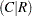LAMDAS

Lambda symmetric

LAMRC

Lambda asymmetric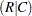LGOR

Adjusted logit odds ratio (tables)

CMH, CMH1, or CMH2

LGRRC1

Adjusted column 1 logit relative risk

CMH, CMH1, or CMH2

LGRRC2

Adjusted column 2 logit relative risk

CMH, CMH1, or CMH2

LRCHI

Likelihood-ratio chi-square

MCNEM

McNemar’s test (tables)

MEASURES

Gamma, Kendall’s tau-, Stuart’s tau-,

Somers’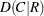and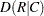, Pearson and

Spearman correlations, lambda asymmetricand, lambda symmetric,

uncertainty coefficientsand,

symmetric uncertainty coefficient;

odds ratio and relative risks (tables)

MHCHI

Mantel-Haenszel chi-square

MHOR | COMOR

CMH, CMH1, or CMH2

(tables)

MHRRC1

Adjusted column 1 Mantel-Haenszel relative risk

CMH, CMH1, or CMH2

MHRRC2

Adjusted column 2 Mantel-Haenszel relative risk

CMH, CMH1, or CMH2

N

Number of nonmissing observations

NMISS

Number of missing observations

OR

Odds ratio (tables)

PCHI

Chi-square goodness-of-fit test for one-way tables,

Pearson chi-square for two-way tables

PCORR

Pearson correlation coefficient

PHI

Phi coefficient

PLCORR

Polychoric correlation coefficient

RDIF1

Column 1 risk difference (row 1 - row 2)

RDIF2

Column 2 risk difference (row 1 - row 2)

RELRISK

Odds ratio and relative risks (tables)

RISKDIFF

Risks and risk differences (tables)

RISKDIFF1

Column 1 risks and risk difference

RISKDIFF2

Column 2 risks and risk difference

RRC1

Column 1 relative risk

RRC2

Column 2 relative risk

RSK1

Column 1 risk, overall

RSK11

Column 1 risk, for row 1

RSK12

Column 2 risk, for row 1

RSK2

Column 2 risk, overall

RSK21

Column 1 risk, for row 2

RSK22

Column 2 risk, for row 2

SCORR

Spearman correlation coefficient

SMDCR

Somers’SMDRC

Somers’STUTC

Stuart’s tau-TREND

Cochran-Armitage test for trend

TSYMM

Bowker’s test of symmetry

U

Symmetric uncertainty coefficient

UCR

Uncertainty coefficientURC

Uncertainty coefficientWTKAP

Weighted kappa coefficient

Footnotes

1. CHISQ computes Fisher’s exact test fortables. Use the FISHER option to compute Fisher’s exact test for general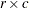tables.